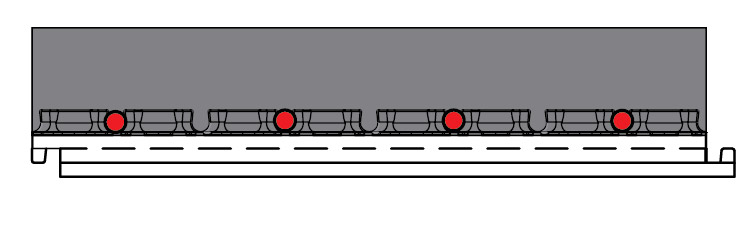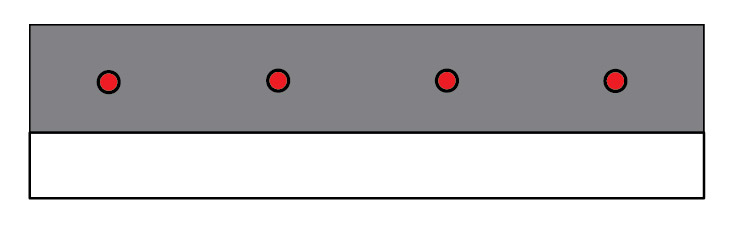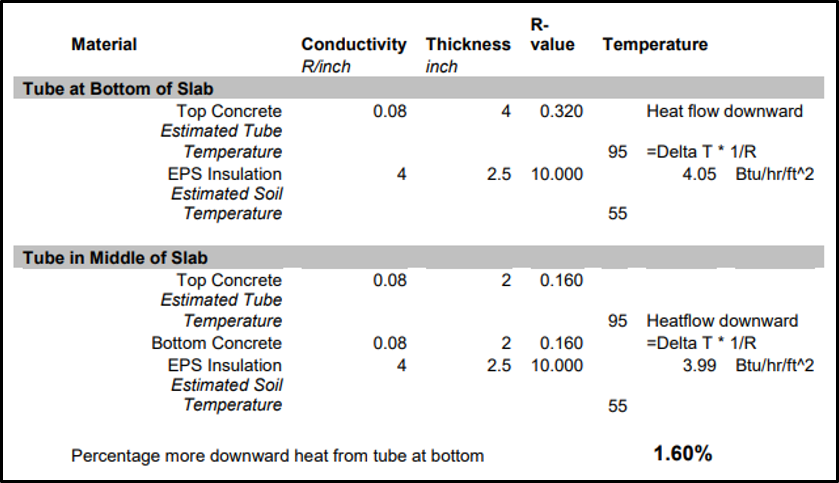###### Radiant Heat Floors for Health and Comfort
August 10, 2020###### Radiant Floor Rough-ins For Your Slab: A Simple Upgrade For Your Reno or Addition
November 13, 2020
Share this story!

Radiant Heat Flooring (RHF) panel systems such as Heat-Sheet save up to 4 times in pipe installation labor. That’s how much faster it is to walk the tubing into place without bending down to staple it.

However, using these panels means placing the tubing at the slab’s bottom rather than the middle.Heating Tubing At The Bottom Of The Slab

And when you lay RHF tubing beneath the slab, increased downward heat loss is a worry for some. After all, you’re moving the heat source closer to the cold soil and eliminating the few concrete inches that separate the two.

But as it turns out, the incremental heat-loss is actually trivial. This post will address this downward heat-loss using simple principles and calculations, as explained by one of Canada’s leading building envelope scientists, Dr. John Straube.

### First, How Does Heat Flow?

Heat always flows from hot to cold. You can calculate its flow rate, or “thermal conductance,” by:

1. Subtracting the material temperature from the heat source temperature; then
2. Dividing 1 by the R-value, or “thermal resistance” of the material through which heat flows; next
3. Multiplying the quotient by the temperature difference

The thermal conductance hinges on 2 factors:

• the ease of flowing through the surrounding material, and
• the temperature difference between the source and the surrounding material

So, to increase the heat flow rate, you must widen this temperature difference and direct heat through a conductive material.

### So How Does Heat Flow From Radiant Tubing?

As a radiant tube warms up, the heat leaving it flows in all directions through the cooler concrete. After a short while, lateral flow weakens as the concrete encasing the tubes gets hot, and the temperature difference fades. The heat travels up to the surface, and down, through the insulation, to the cool soil beneath the slab.

The rate of upward heat flow, which we want, and downward escape, which we try to curb, depends on where the tubing runs.

### Situation #1 – Tubing Located in the Middle of the Slab

Let’s assume a 4-inch slab with Pex tubing running through its center. In this case, heat has to traverse 2 inches of concrete above the tubes, and 2 inches below, before it hits the insulation.

Concrete is an excellent conductor, with a modest R-value of only 0.08 per inch. EPS, on the other hand, is a stellar insulator, giving us R-4.0 per inch. Let’s use the thermal conductance formula we’ve just discussed to figure out how much heat escapes to the soil beneath the insulation. With a tube temp of 95°F, and a soil temp of 55°F, here are the constant values for the formula:

• Temperature difference = 95°F-55°F = 40°F
• Concrete R-value = 0.08 x 2 inches = R-0.160
• 5-inch EPS board R-value = R-4 x 2.5 inches = R-10.000
• Total R-value = R-10.000 + R-0.160 = R-10.160

Now, we can figure out the downward heat flow is for this tube location. Remember the formula: Delta T x 1/R.

###### Downward heat flow = 40 x 1/10.160 = 3.937 Btu/hr/ft2

With this value on hand, it’s time to see how the rate of heat’s downward flow compares in a bottom-of-slab location, as you’d have it with Heat-Sheet.Tubing Located In The Middle Of A Slab

### Situation #2 – Tubing Located at the Bottom of Slab Location

Let’s walk the same Pex tubing into a Heat-Sheet panel, fixed in place at the bottom of the concrete slab, and heat it to 95F. As the example above shows, sideways propagation is quick-lived, even more so with EPS nodules between the tubing.

Right below the tubes is a sheet of EPS with a substantial thermal resistance of R-4 per inch. As it seeks the path of least resistance, heat has nowhere to go but up.

Now, the same formula will show us how much heat gets wasted when the tubes run at the bottom of a slab. Here are the values for this calculation:

• Temperature difference = 95F-55F = 40°F
• 5-inch EPS board R-value = R-4 x 2.5 inches = R-10.000
• Total R-value = R-10.000
• Downward heat flow = 40 x 1/10.000 = 4.000 Btu/hr/ft2Heating Tubing At The Bottom Of The Slab

So there we have it. With all else unchanged, tubing located at the bottom of a concrete slab allows 0.063 BTU/hr/ft2 or just 1.02% more heat to escape downward instead of going up.

That’s a staggeringly low number, considering that the level of concrete conductivity may vary by as much as 10%, depending on the aggregate.Heating Tube Placement

### Wrapping it up

By using Heat-Sheet, you’ll lose only 1.02% of the heat to downward flow.

In contrast, by simply choosing the wrong concrete mix, you can lose up to 10 times more.

The labor-saving benefits of a system like Heat-Sheet far outweigh the minuscule incremental heat loss.